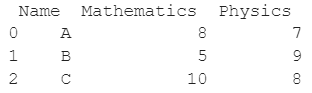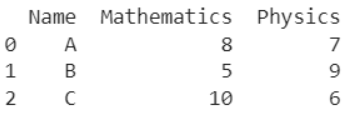# How To Set The Value For Particular Cell In Pandas DataFrame?

A DataFrame is the primary data structure of the Pandas library and is commonly used for storing and working with tabular data. A common operation that could be performed on such data is setting the value of a particular cell in an existing DataFrame in order to add more information to the data.

To start working with Pandas, we first need to import it to Python code:

### Python 3 Code :

``import pandas as pd``

## Running Example

Let us understand this operation with the help of an example. Consider the following DataFrame containing 3 students with names A, B, and C and their corresponding marks (out of 10) for two subjects, Mathematics and Physics.Code snippet for generating the above DataFrame :

### Python 3 Code :

``````import pandas as pd

# Dictionary for our data
data = {'Name' : ['A', 'B', 'C'], 'Mathematics' : [8, 5, 10], 'Physics' : [7, 9, 8]}

# DataFrame for the dictionary
df = pd.DataFrame(data)

# Printing the DataFrame
print(df)``````

Here, data is a dictionary we created to initialize the DataFrame. For this, we use the DataFrame() function of the Pandas library which takes the dictionary as an argument and returns the required DataFrame.

Now let us say that for some reason, we want to change the marks of the student named ‘C’ for the subject Physics from 8 to 6. The resulting DataFrame will look as follows :Let us look at different ways of performing this operation on a given DataFrame :

## Set The Value For Particular Cell In Pandas DataFrame Using the DataFrame.loc property

In this method, we use the DataFrame.loc property to access the cell for which the value needs to be set.

We do this by passing the first argument as the index of the row coordinate of the desired cell, here 2, and the second argument as the label of the column coordinate for this particular cell, here 'Physics'.

The DataFrame.loc property returns a reference to the desired cell and the value can then be set by simple assignment, here 6.

Let us look at the code and corresponding output for this method.

### Python 3 Code :

``````import pandas as pd

# Dictionary for our data
data = {'Name' : ['A', 'B', 'C'], 'Mathematics' : [8, 5, 10], 'Physics' : [7, 9, 8]}

# DataFrame for the dictionary
df = pd.DataFrame(data)

# Setting the marks for Physics of the student named 'C' to 6
df.loc[2,'Physics'] = 6

# Printing the resulting DataFrame
print(df)``````

Output :## Set The Value For Particular Cell In Pandas DataFrame Using the DataFrame.at property

In this method, we use the DataFrame.at property to access the cell for which the value needs to be set.

We do this by passing the first argument as the index of the row coordinate of the desired cell, here 2, and the second argument is the label of the column coordinate for this particular cell, here ‘Physics’.

The DataFrame.at property returns a reference to the desired cell and the value can then be set by simple assignment, here 6.

For this application, the DataFrame.at property is similar to DataFrame.loc property.

Let us look at the code and corresponding output for this method.

### Python 3 Code :

``````import pandas as pd

# Dictionary for our data
data = {'Name' : ['A', 'B', 'C'], 'Mathematics' : [8, 5, 10], 'Physics' : [7, 9, 8]}

# DataFrame for the dictionary
df = pd.DataFrame(data)

# Setting the marks for Physics of the student named 'C' to 6
df.at[2,'Physics'] = 6

# Printing the resulting DataFrame
print(df)``````

Output :## Set The Value For Particular Cell In Pandas DataFrame Using the DataFrame.iloc property

In this method, we use the DataFrame.iloc property to access the cell for which the value needs to be set.

We do this by passing the first argument as the index of the row coordinate of the desired cell, here 2, and the second argument as the index of the column coordinate for this particular cell, here 2.

The DataFrame.iloc property returns a reference to the desired cell and the value can then be set by simple assignment, here 6.

Let us look at the code and corresponding output for this method.

### Python 3 Code :

``````import pandas as pd

# Dictionary for our data
data = {'Name' : ['A', 'B', 'C'], 'Mathematics' : [8, 5, 10], 'Physics' : [7, 9, 8]}

# DataFrame for the dictionary
df = pd.DataFrame(data)

# Setting the marks for Physics of the student named 'C' to 6
df.iloc[2,2] = 6

# Printing the resulting DataFrame
print(df)``````

Output :## Set The Value For Particular Cell In Pandas DataFrame Using the DataFrame.iat property

In this method, we use the DataFrame.iat property to access the cell for which the value needs to be set.

We do this by passing the first argument as the index of the row coordinate of the desired cell, here 2, and the second argument as the index of the column coordinate for this particular cell, here 2.

The DataFrame.iat property returns a reference to the desired cell and the value can then be set by simple assignment, here 6.

For this application, the DataFrame.iat property is similar to DataFrame.iloc property.

Let us look at the code and corresponding output for this method.

### Python 3 Code :

``````import pandas as pd

# Dictionary for our data
data = {'Name' : ['A', 'B', 'C'], 'Mathematics' : [8, 5, 10], 'Physics' : [7, 9, 8]}

# DataFrame for the dictionary
df = pd.DataFrame(data)

# Setting the marks for Physics of the student named 'C' to 6
df.iat[2,2] = 6

# Printing the resulting DataFrame
print(df)``````

Output :## Conclusion

In this topic, we have learned to set the value of a particular cell in an existing DataFrame, following a running example of test scores of students in different subjects, thus giving us an intuition of how this concept could be applied in the real-world situations. Feel free to reach out to [email protected] in case of any suggestions.

Trending